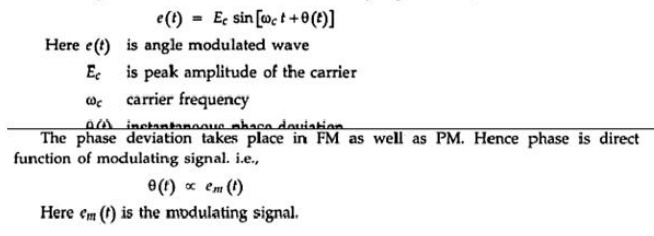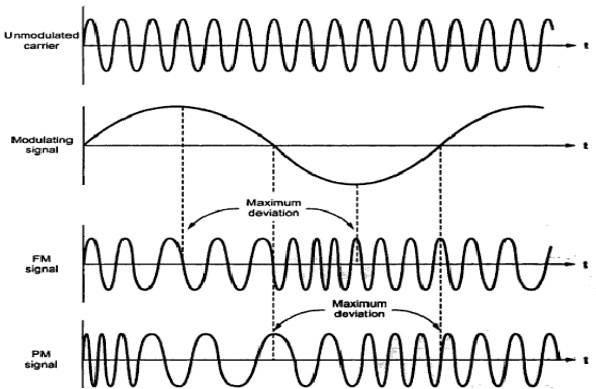Home | | Analog and Digital Communication | The Concept of Angle Modulation and Its Waveform, Frequency and Phase Modulation

# The Concept of Angle Modulation and Its Waveform, Frequency and Phase Modulation

We know that amplitude, frequency or phase of the carrier can be varied by the modulating signal. Amplitude is varied in AM. When frequency or phase of the carrier is varied by the modulating signal, then it is called angle modulation. There are two types of angle modulation.

THE CONCEPT OF ANGLE MODULATION AND ITS WAVEFORM, FREQUENCY AND PHASE MODULATION:

Angle Modulation

Definition

We know that amplitude, frequency or phase of the carrier can be varied by the modulating signal. Amplitude is varied in AM. When frequency or phase of the carrier is varied by the modulating signal, then it is called angle modulation. There are two types of angle modulation.

1. Frequency Modulation: When frequency of the carrier varies as per amplitude variations of modulating signal, then it is called Frequency Modulation(FM). Amplitude carrier remains constant.

2. Phase Modulation: When phase of the carrier varies as per amplitude variations of modulating signal, then it is called Phase Modulation (PM). Amplitude of the modulated carrier remains constant.

The angle modulated wave is mathematically expressed as,FM and PM Waveforms

Fig. 2.1.1 shows the waveforms of FM and PM.

In this figure following observations can be noted:

(i) For FM signal, the maximum frequency deviation takes place when modulating signl is at positive and negative peaks.

(ii) For PM signal the maximum requency deviation takes place near zero crossings o the modulating signal.

(iii)  Both FM and PM waveforms are identical except the phase shit.

(iv)   From modulated waveform it is difficult to know, whether the modulation is FM or PM.The expression for frequency deviation, phase deviation and modulation index in angle modulation.Thus modulation index of PM signal is ditectly proportional to peak modulating voltage. And it's unit is radians.

Modulation index for FM:Thus modulation index of M is unities ratio. From above equation and equation 2.1.14, note that the modulation index is differently defined for M and PM signals.

Percentage modulation:

For angle modulation, the percentage modulation is given as the ratio of actual frequency derviation to maximum allowable frequency deviation. i.e.,Study Material, Lecturing Notes, Assignment, Reference, Wiki description explanation, brief detail
Analog and Digital Communication : The Concept of Angle Modulation and Its Waveform, Frequency and Phase Modulation |